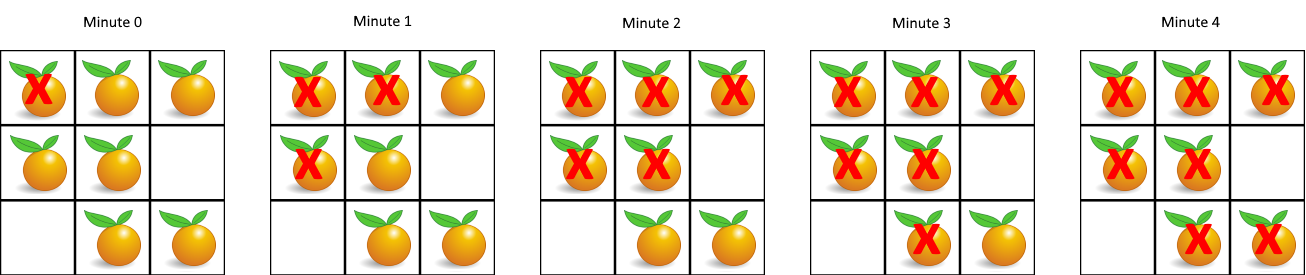# LeetCode: 994. 腐烂的橘子¶

## 1、题目描述¶输入：[[2,1,1],[1,1,0],[0,1,1]]



输入：[[2,1,1],[0,1,1],[1,0,1]]



输入：[[0,2]]



## 2、解题思路¶

• 可以使用广度优先搜索
• 从腐烂的橘子为起点，每次感染周围4个橘子，直到所有能被感染的橘子都被感染为止

• 首先将橘子的坐标与感染时间放进去
• 每次取出一个感染橘子，去感染周围的橘子，将新感染的放入队列，时间+1
• 依次判断，直到队列为空
• 此时判断是否存在未感染的橘子，存在则返回-1，不存在返回最后一个被感染的橘子的时间
from collections import deque
class Solution:
def orangesRotting(self, grid: List[List[int]]) -> int:
R, C = len(grid), len(grid)
q = deque()

for r, row in enumerate(grid):
for c, num in enumerate(row):
if num == 2:
# 记录腐烂的橘子位置以及腐烂需要的时间
q.append((r, c, 0))
total = 0
while q:
r, c, total = q.popleft()
for row, col in ((r - 1, c), (r + 1, c), (r, c - 1), (r, c + 1)):
if 0 <= row < R and 0 <= col < C:
if grid[row][col] == 1:
q.append((row, col, total + 1))
grid[row][col] = 2
if any((1 in row) for row in grid):
return -1